# 如何分析随温度变化的特征频率

2017年 5月 22日

### 矩形梁示例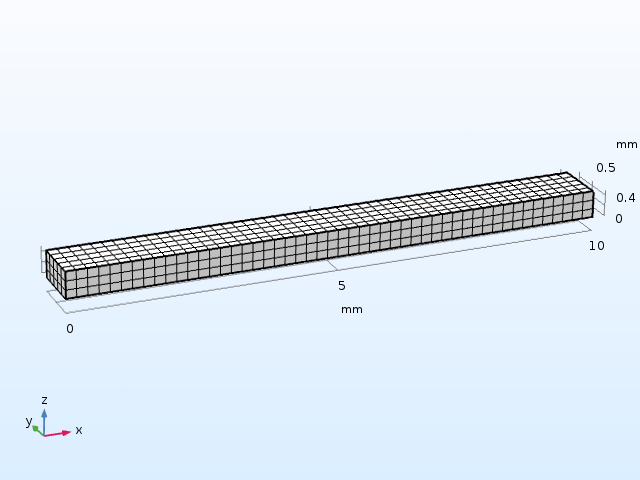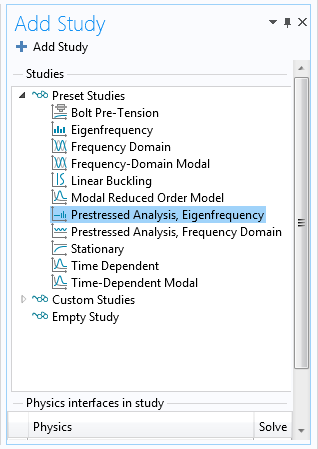1. 稳态 研究步骤，计算热膨胀引起的位移和应力
2. 特征频率 研究步骤，其中需要用到上一步计算的解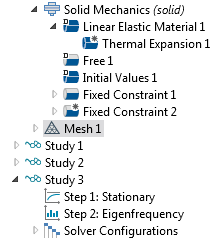“模型开发器”树中显示的两个研究步骤。

1. 双端固支梁
2. 悬臂梁，其一端固定，另一端自由活动

= 10K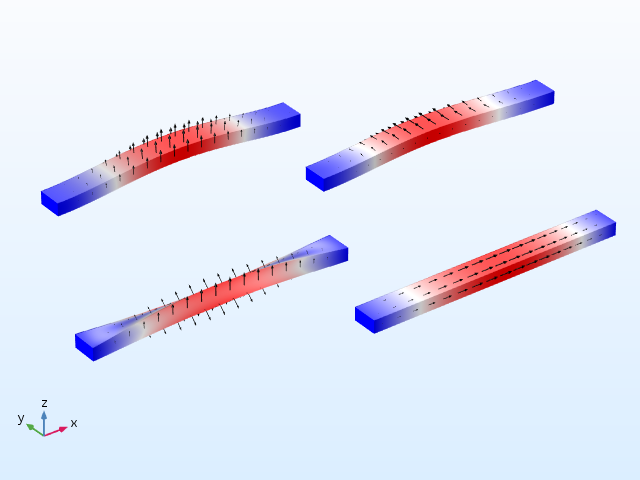= 10K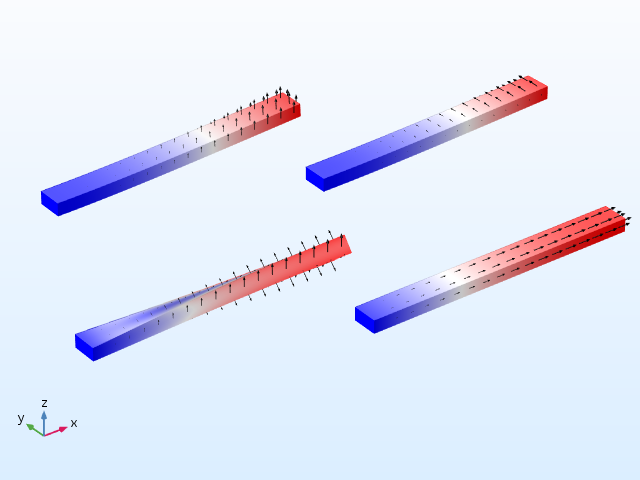### 研究应力软化效应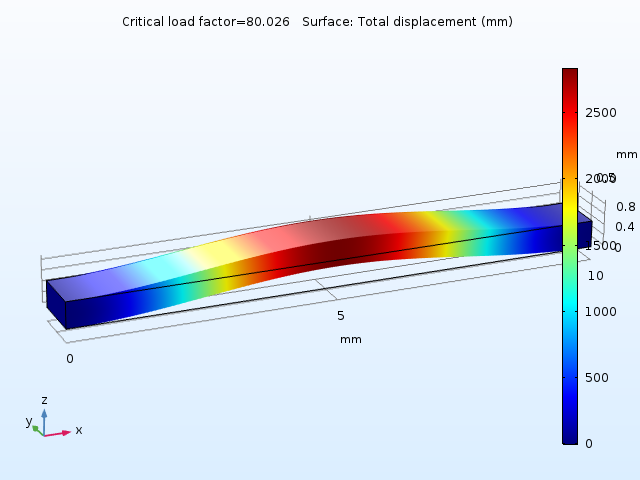1-\frac{1}{80}
= 0.9875

### 评估几何变化如何影响频率

f_b \propto \frac{1}

{L^2}
\sqrt{\frac

{EI}
{\rho A}}

f_t \propto \frac{1}

{L} \sqrt{\frac{GK}{\rho J}}

f_a \propto \frac{1}{L}
\sqrt{\frac{E}
{\rho}}

• I = 弯曲轴周围的面积惯性矩
• G = 剪切模量
• K = 扭转模量
• J = 绕梁轴的极惯性矩

\begin{align} L& =L_0(1+\epsilon_x)  \\\end{align}
\begin{align} A& = ab = a_0b_0(1+\epsilon_y)(1+\epsilon_z) \\\end{align}
\begin{align} I_y& = \frac{ab^3}{12} = \frac{a_0b_0^3}{12}
(1+\epsilon_y)(1+\epsilon_z)^3 \\\end{align}
\begin{align} I_z& = \frac{ba^3}{12} = \frac{b_0a_0^3}{12}
(1+\epsilon_z)(1+\epsilon_y)^3 \\\end{align}
\begin{align} K& = \frac{ab^3}{3}F_1(a/b) \approx \frac{a_0b_0^3}{3}
F_1(a_0/b_0)(1+\epsilon_y)(1+\epsilon_z)^3 \\\end{align}
\begin{align} J& =\frac{ab^3+ba^3}{12}=\frac{a_0b_0^3(1+\epsilon_y)(1+\epsilon_z)^3+b_0a_0^3(1+\epsilon_z)(1+\epsilon_y)^3}{12}
\\\end{align}

\rho = \frac{\rho_0}

{(1+\epsilon_x)(1+\epsilon_y)(1+\epsilon_z)}

\frac{f_{b,z}}{f_{b0,z}} = \sqrt{\frac

{(1+\epsilon_y)(1+\epsilon_z)^3}
{(1+\epsilon_x)^3}} \approx 1-\frac{3\epsilon_x}

{2}+\frac{\epsilon_y}{2}
+\frac{3\epsilon_z}

{2}

\frac{f_{b,y}}{f_{b0,y}} = \sqrt{\frac{(1+\epsilon_z)(1+\epsilon_y)^3}{(1+\epsilon_x)^3}} \approx 1-\frac{3\epsilon_x}{2}
+\frac{3\epsilon_y}{2}+\frac{\epsilon_z}{2}

\frac{f_a}{f_{a0}} = \sqrt{\frac

{(1+\epsilon_y)(1+\epsilon_z)}
{(1+\epsilon_x)}} \approx 1-\frac{\epsilon_x}

{2}+\frac{\epsilon_y}{2}
+\frac{\epsilon_z}

{2}

\frac{f_{t}}{f_{t0}} = \sqrt{\frac{5(1+\epsilon_z)(1+\epsilon_y)^3}{(1+\epsilon_x)((1+\epsilon_z)^2+ 4(1+\epsilon_y)^2))}} \approx 1-\frac{\epsilon_x}{2}
-\frac{3\epsilon_y}{10}+\frac{6\epsilon_z}{5}

### 约束建模的效果分析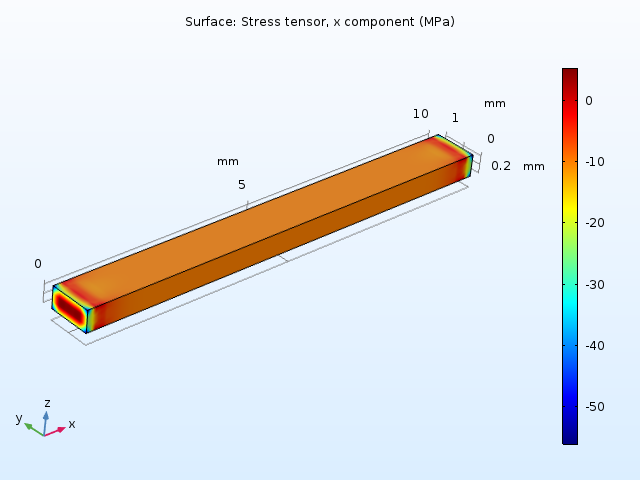10K 温升引起的双端固支梁轴向应力。

1. 在预期仅经历体积变化的组件中可能发生应力硬化
2. 由于横向位移受到限制（如上例所示），横截面尺寸不再是恒定的### 包含材料数据中的温度依存性

COMSOL Multiphysics “材料库”中的大多数材料都具有温度相关的材料属性。在本例中，您可以通过以下步骤手动将线性温度相关性添加到杨氏模量：

1. 基本 属性组的设置中，选择模型输入 下的温度
2. 单击添加 以查看要使用的变量名称 T
3. 写出杨氏模量的表达式，它是 的函数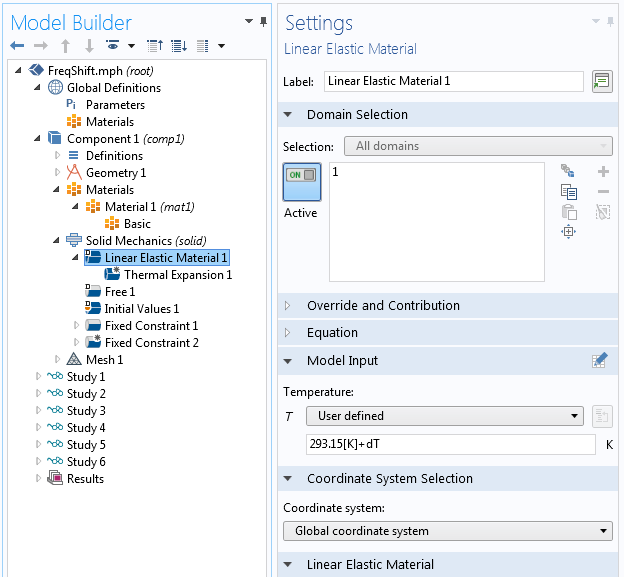\frac{f_{b,z}}{f_{b0,z}} \approx 1+ \left (-\frac{3\alpha_x}{2}+\frac{\alpha_y}{2}+\frac{3\alpha_z}{2}+\frac{\beta}{2} \right)\Delta T
\frac{f_{b,y}}{f_{b0,y}} \approx 1 + \left (-\frac{3\alpha_x}{2}+\frac{3 \alpha_y}{2}+\frac{\alpha_z}{2}+\frac{\beta}{2} \right)\Delta T
\frac{f_a}{f_{a0}} \approx 1 + \left (-\frac{\alpha_x}{2}+\frac{\alpha_y}{2}+\frac{\alpha_z}{2}+\frac{\beta}{2} \right)\Delta T
\frac{f_{t}}{f_{t0}} \approx 1 + \left (\frac{3\alpha_x}{2}\frac{3 \alpha_y}{10}
+\frac{6\alpha_z}{5}\frac{\beta}{2} \right)\Delta T

\frac{f_{b,z}}{f_{b0,z}} = \frac{f_{b,y}}{f_{b0,y}} = \frac{f_a}{f_{a0}} \approx 1+ \left (\frac{\alpha}{2}\frac{\beta}{2} \right)\Delta T
\frac{f_{t}}{f_{t0}} \approx 1 + \left (-\frac{3\alpha}{5}
+\frac{\beta}{2}
\right)\Delta T

### 关于数值精度的说明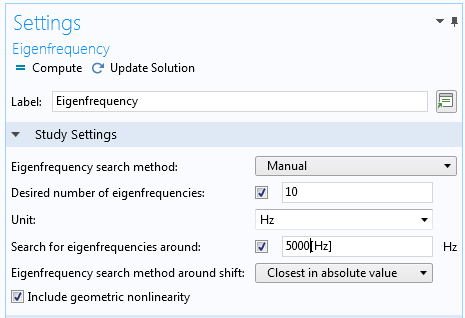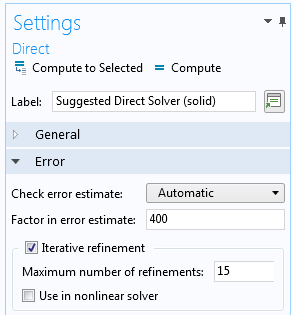### 几何非线性下处理非弹性应变的理论变化

1+\epsilon_x = 1.3
1+\epsilon_y = 1.6
1+\epsilon_z = 1.9

### 其他资源

• 阅读以下关于结构力学分析的博客文章：
• 请参阅“发布亮点”页面上的“结构力学模块”更新

#### 评论 (2)

##### 留言##### 霄砻 简
2022-12-13##### hao huang
2022-12-15 COMSOL 员工